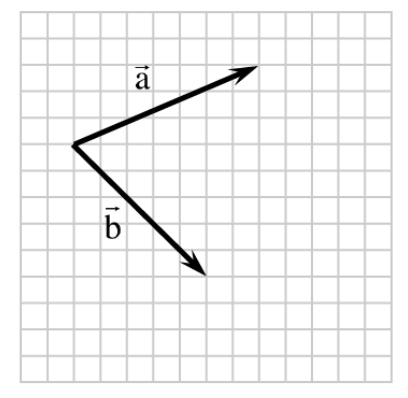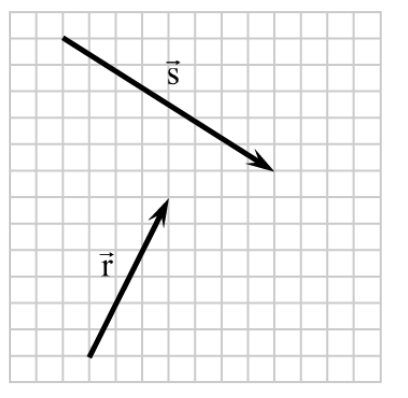### Home > PC3 > Chapter 6 > Lesson 6.2.3 > Problem6-110

6-110.

Use the diagrams below to complete the following problems.To sketch a negative vector, interchange the 'head' and tail' of the given vector.
To add vectors, place the tail of the second vector at the head of the first vector.
Then sketch the resultant vector by connecting the initial point of the first vector to the final point of the second vector.

1. Copy $\vec { \text{a} }$ and $\vec { \text{b} }$ onto graph paper. Draw $-\vec { \text{b} }$. Then add $\vec { \text{a} }$ and $-\vec { \text{b} }$. Explain why you can label your answer $\vec { \text{a} } - \vec { \text{b} }$.

2. Copy $\vec { \text{r} }$ and $\vec { \text{s} }$ onto graph paper. Then draw $\vec { \text{r} } - \vec { \text{s} }$.